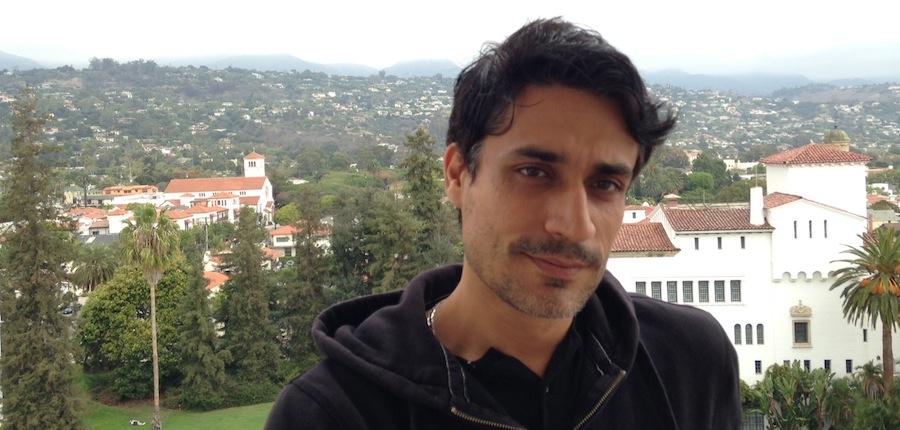### Hamid Hezari# Hamid Hezari

Associate Professor
Department of Mathematics
UC Irvine

Office: Rowland Hall 510J
E-mail: my last name at uci dot edu

I got my PhD from Johns Hopkins University in 2009. From 2009-2012 I was a CLE Moore instructor at MIT, and I spent the fall of 2010 as a postdoc at the MSRI program on inverse problems. I am currently an associate professor at University of California, Irvine.

My research is in analysis and partial differential equations. I am especially interested in semiclassical/microlocal analysis and its applications in PDE and mathematical physics, in particular spectral geometry and quantum chaos.

# Publications and Preprints

29. Upper bounds on the size of nodal sets for Gevrey and quasianalytic Riemannian manifolds, submitted, arXiv-pdf

28. One can hear the shape of ellipses of small eccentricity (with Steve Zelditch), To appear in Annals of Mathematics, January 2023 issue, Vol. 197, No. 1, arXiv-pdf

27. Centrally symmetric analytic plane domains are spectrally determined in this class (with Steve Zelditch), submitted, arXiv-pdf

26. The Dirichlet isospectral problem for trapezoids (with Zhiqin Lu and Julie Rowlett), Journal of Mathematical Physics, Vol. 62, 2021, arXiv-pdf

25. Eigenfunction asymptotics and spectral rigidity of the ellipse (with Steve Zelditch), Journal of Spectral Theory, Vol. 12, special issue in memory of M. Shubin, 2022, arXiv-pdf

24. On a property of Bergman kernels when the Kähler potential is analytic (with Hang Xu), Pacific Journal of Mathematics, Vol. 313, No. 2, 2021, arXiv-pdf

23. Equidistribution of toral eigenfunctions along hypersurfaces (with Gabriel Rivière), Revista Mathemática Iberoamericana, Vol. 36, No. 2, 2019, arXiv-pdf

﻿

22. Quantitative upper bounds for Bergman kernels associated to smooth Kähler potentials (with Hang Xu), Mathematical Research Letters, Vol. 27, No. 3, 2020, arXiv-pdf

21. Off-diagonal asymptotic properties of Bergman kernels associated to analytic Kähler potentials (with Zhiqin Lu and Hang Xu), International Mathematics Research Notices, Vol. 2020, Issue 8, 2020, arXiv-pdf

20. An appendix of Dynamical spectral rigidity among ℤ2-symmetric strictly convex domains close to a circle by De Simoi, Kaloshin, and Wei (joint with the authors), Annals of Mathematics, Vol. 186, No. 1, 2017, arXiv-pdf

19. Robin spectral rigidity of nearly circular domains with a reflectional symmetry, Communications in Partial Differential Equations, Vol. 42, No. 9, 2017, arXiv-pdf

18. Quantum ergodicity and Lp norms of restrictions of eigenfunctions, Communications in Mathematical Physics, ﻿Vol. 357, No. 3, 2018, arXiv-pdf

17. Inner radius of nodal domains of quantum ergodic eigenfunctions, Proceedings of American Mathematical Society, ﻿Vol. 146, No. 11, 2018, arXiv-pdf

16. Applications of small scale quantum ergodicity in nodal sets, Analysis and PDE, ﻿Vol. 11, No. 4, 2018, arXiv-pdf

15. The Neumann isospectral problem for trapezoids (with Julie Rowlett and Zhiqin Lu), Annales Henri Poincaré , ﻿Vol. 18, No. 12, 2017, arXiv-pdf

14. Quantitative equidistribution properties of toral eigenfunctions (with Gabriel Rivière). Journal of Spectral Theory , Vol. 7, No. 2, 2017, arXiv-pdf

13. Lp norms, nodal sets, and quantum ergodicity (with Gabriel Rivière). Advances in Mathematics, Vol. 290, 2016, arXiv-pdf

12. Asymptotic expansion of the Bergman kernel via perturbation of the Bargmann-Fock model (with Casey Kelleher, Shoo Seto, and Hang Xu). Journal of Geometric Analysis, Vol. 26, No. 4, 2016, arXiv-pdf

11. Completeness of boundary traces of eigenfunctions (with Xioalong Han, Andrew Hassell, and Steve Zelditch). Proceedings of the London Mathematical Society, Vol. 111, No. 3, 2015, arXiv-pdf

10. A Fulling-Kuchment theorem for the harmonic oscillator (with Victor Guillemin). Inverse Problems, Vol. 28, No. 4, 2012, arXiv-pdf

9. Inverse problems in spectral geometry, a survey on inverse spectral problems (with Kiril Datchev). Inverse Problems and Applications: Inside Out II, MSRI Publications, No. 60, 2012, arXiv-pdf

8. A natural lower bound for the size of nodal sets (with Christopher Sogge). Analysis and PDE, Vol. 5, No. 5, 2012, arXiv-pdf

7. Lower bounds for volumes of nodal sets: an improvement of a result of Sogge-Zelditch (with Zuoqin Wang). AMS Special Issue on Spectral Geometry, 2012, arXiv-pdf

6. Resonant uniqueness of radial semiclassical Schrödinger operators (with Kiril Datchev). Applied Mathematics Research eXpress, Vol. 2012, No. 1, 2012, arXiv-pdf

5. Spectral uniqueness of radial semiclassical Schrödinger operators (with Kiril Datchev and Ivan Ventura ). Mathematical Research Letters, Vol. 18, No. 3, 2011, arXiv-pdf

4. Cspectral rigidity of the ellipse (with Steve Zelditch). Analysis and PDE, Vol. 5, No. 5, 2012, arXiv-pdf

3. Inverse spectral problems for (Z/2Z)n-symmetric domains in Rn (with Steve Zelditch). GAFA, Vol. 20, No. 1, 2010, arXiv-pdf

2. Inverse spectral problems for Schrödinger operators. Communications in Mathematical Physics, No. 3, 2009, arXiv-pdf

1. Complex zeros of eigenfunctions of 1-dimensional Schrödinger Operators. International Mathematics Research Notices, No.3, 2008, arXiv-pdf

# Teaching at UC Irvine

Math 140B: Elementary Analysis, Spring 2021

Math 140A: Elementary Analysis, Winter 2021 and Spring 2021

Math 3D: Ordinary Differential Equations (Lec A and B), Spring 2020

Math 112B: Introduction to Partial Differential Equations, Winter 2020

Math 2E: Multivariable Calculus, Winter 2019

Math 112B: Introduction to Partial Differential Equations, Winter 2019

Math 147: Complex Analysis, Spring 2018 and Fall 2018

Math 3D: Ordinary Differential Equations, Fall 2017

Math 112A: Introduction to Partial Differential Equations, Fall 2017

Math 140B: Elementary Analysis, Spring 2017

Math 3D: Ordinary Differential Equations, Winter 2017

Math 3D: Ordinary Differential Equations, Fall 2017

Math 112A: Introduction to Partial Differential Equations, Fall 2017

Math 140B: Elementary Analysis, Spring 2017

Math 3D: Ordinary Differential Equations, Winter 2017

Math 2E: Multivariable Calculus, Fall 2016

Math 112A: Introduction to Partial Differential Equations, Fall 2016

Math 2D: Multivariable Calculus, Winter 2016

Math 296: Topics course on Microlocal Analysis, Fall 2015

Math 13: Introduction to Abstract Mathematics, Summer 2015

Math 2D: Multivariable Calculus, Summer 2015

Math 3A: Linear Algebra, Spring 2015

Math 296: Harmonic Analysis, Winter 2015

Math 2D: Multivariable Calculus, Fall 2014

Math 205ABC: Introduction to Graduate Analysis, Fall 2013, Winter 2014, Spring 2014

Math 117: Dynamical Systems, Winter 2013

Math 114: Complex Analysis, Fall 2012

Math 118: Theory of Differential Equations, Fall 2012